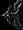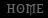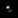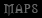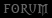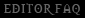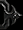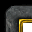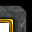# JASS: Introduction

Tutorial By Vexorian

Introduction to JASS

This is supposed to be a brief introduction to JASS and programming, you might or might not need GUI (triggers) knowledge, the point of it is to make you ready to start reading other tutorials, open source JASS scripts and even the manual at http://jass.sourceforge.net/doc/ so you start the learning curve.

If you can already handle JASS this is not the tutorial for you. Also if you already know a programming language, the manual at http://jass.sourceforge.net/doc/ should be enough for you.

My target is to make a practical JASS course, since there are already plenty of theorical tutorias. I will purposely skip some stuff, remember that this is just to start in JASS, and after that you will have to see Open source JASS scripts and other Tutorials.

The only thing I will require for you is that you should already know how to extract files from a MPQ , search for that in the forums or google. But I don't want to ellaborate on that.

The first step is to open World Editor, create a new map and then open the trigger editor, select the trigger 'Map initialization' and you will most probably see this:

Melee Initialization Events Map initialization Conditions Actions Melee Game - Limit Heroes to 1 per Hero-type (for all players) Melee Game - Give trained Heroes a Scroll of Town Portal (for all players) Melee Game - Use melee time of day (for all players) Melee Game - Set starting resources (for all players) Melee Game - Remove creeps and critters from used start locations (for all players) Melee Game - Create starting units (for all players) Melee Game - Run melee AI scripts (for computer players) Melee Game - Enforce victory/defeat conditions (for all players)

Now we will have to set the battle field ready for battle, change the Event to A Player skips a cinematic, remove the actions and replace them with a text message action. Rename the trigger to JASS test.

JASS test Events Player - Player 1 (Red) skips a cinematic sequence Conditions Actions Game - Display to (All players) the text: This is a message

Time to get started, go to the edit menu and Convert It to custom text. press OK.

```function Trig_JASS_test_Actions takes nothing returns nothing
call DisplayTextToForce( GetPlayersAll(), "TRIGSTR_004" )
endfunction

//===========================================================================
function InitTrig_JASS_test takes nothing returns nothing
set gg_trg_JASS_test = CreateTrigger(  )
call TriggerRegisterPlayerEventEndCinematic( gg_trg_JASS_test, Player(0) )
call TriggerAddAction( gg_trg_JASS_test, function Trig_JASS_test_Actions )
endfunction```

The first obstacle, what happened to the "This is a message" text?

TRIGSTR_004 , reffers to a string in war3map.wts (the map strings) you are able to edit that file with the World Editor's File\Export/Import Strings... commands. But for practical reasons we will forget about that and just use the text we like.

```function Trig_JASS_test_Actions takes nothing returns nothing
call DisplayTextToForce( GetPlayersAll(), "This is a message" )
endfunction

//===========================================================================
function InitTrig_JASS_test takes nothing returns nothing
set gg_trg_JASS_test = CreateTrigger(  )
call TriggerRegisterPlayerEventEndCinematic( gg_trg_JASS_test, Player(0) )
call TriggerAddAction( gg_trg_JASS_test, function Trig_JASS_test_Actions )
endfunction```

Here we will see 2 functions , Trig_JASS_test_Actions and InitTrig_JASS_test.

Each "Trigger" in the Trigger editor comes with a InitTrig_TRIGGERNAME (Spaces are replaced with _) function that is always called at the initialization of the map.

In this case we are talking about the InitTrig_JASS_test function. In the contents of that function we will see these lines:

```    set gg_trg_JASS_test = CreateTrigger(  )
call TriggerRegisterPlayerEventEndCinematic( gg_trg_JASS_test, Player(0) )
call TriggerAddAction( gg_trg_JASS_test, function Trig_JASS_test_Actions )```

For now we will say they are the Setup of a trigger that is saved under the gg_trg_JASS_test variable. A line to highlight is

`    call TriggerAddAction( gg_trg_JASS_test, function Trig_JASS_test_Actions )`

It says that the action function for the gg_trg_JASS_test trigger is Trig_JASS_test_Actions. So for now the only thing we will do is editing the contents of that function , keep the other function with no changes.

We are now ready to start the course!

1. First Saving.

Save the map, it shouldn't give you errors unless you made a major mistake when replacing the contents of the message, double check it.

Most likely the message MUST be between quotes "" .

If it doesn't give you errors, Test the map using the Test Map button.

Now in game press Escape, it should show the "This is a message" text. This is the way we will test JASS for now.

2. Variables Tests

The syntax for making a local variable inside a function is the following:

local <VARIABLE TYPE> <VARIABLE NAME>

Optionally you can initiate the local variable with a value

local <VARIABLE TYPE> <VARIABLE NAME> = value

Variable names should not start with a number, and may only have alpha numeric characters and _

Strings
The first type we will use is string , a string is just text between "". The syntax does not care about what you use inside the string.

We will call the variable "a" .

It is time to say that JASS is case sensitive, so typing A is not the same as typing a , you can't use LOCAL nor Local for the declaration of the variable, you must use local.

```function Trig_JASS_test_Actions takes nothing returns nothing
local string a="This is a message"
call DisplayTextToForce( GetPlayersAll(), a )
endfunction```

In the example we just moved the message to the value of the variable named "a". When we used the variable in the Function that shows the message we didn't put it inside "".

Test the map, it should work the same way it worked before.

You can have multiple variables inside a function, but always make sure the declaration of the variables is before any other line.

```function Trig_JASS_test_Actions takes nothing returns nothing
local string a="This is a message"
local string b="This is other message"
call DisplayTextToForce( GetPlayersAll(), a )
endfunction```

What's the point of having 2 variables if we don't use the second one? Let us copy the Message function call to create another one. Then replace a with b

```function Trig_JASS_test_Actions takes nothing returns nothing
local string a="This is a message"
local string b="This is other message"
call DisplayTextToForce( GetPlayersAll(), a )
call DisplayTextToForce( GetPlayersAll(), b )
endfunction```

When you test the map it will show both messages.

Changing the contents of a variable
To change the value of a variable. We use a set statement.

set <variable name> =

```function Trig_JASS_test_Actions takes nothing returns nothing
local string a="This is a message"
local string b="This is other message"
call DisplayTextToForce( GetPlayersAll(), a )
set a="This is a new value!"
call DisplayTextToForce( GetPlayersAll(), a )
endfunction```

Test the map, the result will be
This is a message
This is a new value!

Concatenation
When using String variables you will have to use concatenation. Concatenation is an operation that puts strings together.

Concatenation happens when you use the + operator between string values.

```function Trig_JASS_test_Actions takes nothing returns nothing
local string a="hell"
local string b="o"
call DisplayTextToForce( GetPlayersAll(), a+b )
endfunction```

The result will show the "hello" message.

You can use concatenation to unify a simple string value with a variable

```function Trig_JASS_test_Actions takes nothing returns nothing
local string a="hell"
call DisplayTextToForce( GetPlayersAll(), a+"o" )
endfunction```

The result is hello as well.

You can concatenate multiple strings.

```function Trig_JASS_test_Actions takes nothing returns nothing
local string a="AA"
call DisplayTextToForce( GetPlayersAll(), "The value of a is "+a+" so live with it!" )
endfunction```

The result is
The value of a is AA so live with it!

Null strings
A null strings might be null or "" .

But a string variable can't be set to null , instead you use "" to reset the string to empty

Arrays
An array is basically a group of variables, that share the same name, but have different indexes. To declare arrays we use:

local (ARRAY_TYPE) array (ARRAY_NAME)

You can't initialize an array when declaring it.

The way to use or change the value of an array is different, instead of just using the name of the variable, you have to use the name of the variable + [index]

Indexes of arrays might go from 0 to 8191.

```function Trig_JASS_test_Actions takes nothing returns nothing
local string array a
set a=" zero "
set a=" one "
call DisplayTextToForce( GetPlayersAll(), "Concatenation with values of arrays: "+a+a )
endfunction```

The result in game is:
"Concatenation with values of arrays: zero one "

We will go back into arrays later.

Important note about variables
Variables must have a value before you access them.

Try this function:

```function Trig_JASS_test_Actions takes nothing returns nothing
local string a
call DisplayTextToForce( GetPlayersAll(), "The value of a is +"a+" so live with it!" )
endfunction```

When you go to the game and press escape, nothing will happen, trying to access the a variable will just halt the trigger.

Integers
Now we will test integer variables, Integers are just numbers without a fractionary part that might be negative.

I2S
This function will convert an integer into a string, usage is I2S(integer)

```function Trig_JASS_test_Actions takes nothing returns nothing
local integer a=34
call DisplayTextToForce( GetPlayersAll(), "Value of a is "+I2S(a) )
endfunction```

The result : Value of a is 34

Integer operators

+ : addition
* : Product
/ : division
- : substraction

As a standard, computer languages and math in general consider product and division before addition and substraction.

(): You can use parenthesis to determine which operation goes first.

Operators can be used between variables, values (constants), and/or functions.

```function Trig_JASS_test_Actions takes nothing returns nothing
local integer a=1
local integer b=2
local integer c=3
local integer d=4
local integer r

set r= a+b+c*d
call DisplayTextToForce( GetPlayersAll(), "1. r= "+I2S(r) )
set r= (a+b+c)*d
call DisplayTextToForce( GetPlayersAll(), "2. r= "+I2S(r) )
set r= a+(b-c)
call DisplayTextToForce( GetPlayersAll(), "3. r= "+I2S(r) )
set r= (a+b+c+d)/5
call DisplayTextToForce( GetPlayersAll(), "4. r= "+I2S(r) )
endfunction```

Result:

1. r= 15
2. r= 24
3. r= 0
4. r= 2

Explanation:
1+2+3*4 = 1+2+12 = 15
(1+2+3)*4 = 6*4 = 24
1+(2-3) = 1 + (-1) = 0
(1+2+3+4) / 5 = 10 / 5 = 2

Division between integers will not round the values.

```function Trig_JASS_test_Actions takes nothing returns nothing
local integer r = 28 / 5
call DisplayTextToForce( GetPlayersAll(), "r= "+I2S(r) )
endfunction```

Result: r= 5

28 / 5 equals to 5.6 , if it rounded the result would be 6 instead of 5

Back to the past
Concanenation between integers works if you first convert them to strings.

```function Trig_JASS_test_Actions takes nothing returns nothing
local integer a = 2
local integer b = 3

call DisplayTextToForce( GetPlayersAll(), I2S(a)+I2S(b) )
endfunction```

The result will be 23.

If you want the result to be an integer, you will have to convert the string back to an integer. For this we use S2I(string) .

```function Trig_JASS_test_Actions takes nothing returns nothing
local integer a = 2
local integer b = 3
local string st= I2S(a)+I2S(b)
local integer new = S2I(st)

set a= new-1

call DisplayTextToForce( GetPlayersAll(), I2S(a) )
endfunction```

The result is 22

Arrays
Integer arrays, or arrays of any type work the same

local (ARRAY_TYPE) array (ARRAY_NAME)

The index of an array between [] (remember?) is an integer, but make sure you don't use negatives there.

```function Trig_JASS_test_Actions takes nothing returns nothing
local integer a = 2
local integer array b
set b[a]   = 5
set b[a+1] = 7
set b[a+2] = b[a+1]-b[a]
set b = b[a+2] + 335
call DisplayTextToForce( GetPlayersAll(), I2S(b[a]) )
call DisplayTextToForce( GetPlayersAll(), I2S(b[a+1]) )
call DisplayTextToForce( GetPlayersAll(), I2S(b[a+2]) )
call DisplayTextToForce( GetPlayersAll(), I2S(b) )
endfunction```

The result is:
5
7
2
337

Reals
Reals are numbers, unlike integers they allow(have) fractionary-decimal parts.

Operators and rules for reals are mostly the same as for integers.

But division will not round them.

We use R2S(real) to convert a real into a string

Integers can be used as reals unless it is a return value (see the functions section)

```function Trig_JASS_test_Actions takes nothing returns nothing
local real r = 28 / 5
call DisplayTextToForce( GetPlayersAll(), "r= "+R2S(r) )
endfunction```

Oops, we have 5.000 as result, what happened?

28 and 5 are integers, and the game reads them an integers, so / between 28 and 5 is an integer division, after that the result (5) is converted to real and it becomes 5.0

Changing some stuff:
```function Trig_JASS_test_Actions takes nothing returns nothing
local real r = 28.0 / 5.0
call DisplayTextToForce( GetPlayersAll(), "r= "+R2S(r) )
endfunction```

The result is 5.600

Note that even if the results are shown with 3 decimal digits, internally the game considers much more digits, it is R2S() which makes the string to only show 3 decimals.

Again:
```function Trig_JASS_test_Actions takes nothing returns nothing
local real r1 = 28.0 / 5
local real r2 = 28 / 5.0
call DisplayTextToForce( GetPlayersAll(), "r1= "+R2S(r1) )
call DisplayTextToForce( GetPlayersAll(), "r2= "+R2S(r2) )
endfunction```

Result:
r1= 5.600
r2= 5.600

For the operation to be done in the "real world", only 1 of the values operated has to be real.

While integers may be used as reals, reals can't be used as integers. To use a real as an integer we use the R2I(real) function.

```function Trig_JASS_test_Actions takes nothing returns nothing
local real a = 28.0 / 5.0
local integer r =R2I(a)
call DisplayTextToForce( GetPlayersAll(), "r= "+I2S(r) )
endfunction```

The result is:
r= 5

R2I does not round.

You can combine integer operations and real operations

```function Trig_JASS_test_Actions takes nothing returns nothing
local integer a=5
local integer b=7
local real c = (a*b) / 34.55

set a = R2I(c) + 5
call DisplayTextToForce( GetPlayersAll(), "a= "+I2S(a) )
endfunction```

The result is going to be 6.

Boolean
Booleans are either true or false values.

There are functions that return boolean values, and there are also comparissions that return boolean values.

There is no B2S function that translates a boolean into a string, you can always make one yourself though. But for this course, I will just talk about booleans, then advance into the statements section where we will use booleans.

Comparisions
== equal to
!= not equal to

They are used with any type, even between booleans. If 2 values match == will return true, and != false, if they don't match == will be false and != true .

< Less than
<= Less than or equal
>= Greater than or equal
> Greater than

These comparisions will only work between integers and reals.

Operators
not: The boolean becomes its opposite

not true will return false
not(true) will return false
not false will return true
not true will return false

and: Both values are true
true and true returns true
false and false returns false
false and true returns false
true and false returns false

or: Any of the values is true
true or true returns true
false or false returns false
false or true returns true
true or false returns true

We will say more about boolean values and variables later.

Other types
There are other types, integer,real, string and boolean are just the native types.

The other types are handle and its descendants, handles are objects like unit, trigger, multiboard.

Declaring and setting variables of these types works the same way as for the other types, And you can use == and != comparisions for them.

For more information about how to use them you'd have to extract scripts\common.j and scripts\blizzard.j from war3patch.mpq , and browse those files with a text editor looking for the type declarations and functions usage. Or you can use the api browser at http://jass.sourceforge.net/doc/ (see the end of the function tests section for more information)

Global Variables
We used local variables on the tests, but the Global variables also exist. A Global variable is not specific for the function and can be used on every function.

To declare a global variable you would have to go the map script, and find the globals declaration section like:

```globals
...
<VARIABLE TYPE> <VARIABLE NAME> [= VALUE]
<ARRAY TYPE> array <ARRAY NAME>
constant <Constant type> <Constant name> = <value>
...
endglobals```

But , there is no (easy) way to edit the map script and keeping the triggers. The globals endglobals would only be used if you are making a map script from scratch, or if it is common.j or blizzard.j

But we are interested into using global variables in JASS scripts inside just the trigger editor. There are many ways of making global variables.

Variable Editor
Inside the trigger editor go to the Edit\Variables... menu command. In the variable editor go to Edit\New variable .

For this test use glob as name and integer as type.

Press ok.

Global variables declared with the variable editor get the udg_ preffix automatically. (udg stands for USER DEFINED GLOBAL)

```function Trig_JASS_test_Actions takes nothing returns nothing
set udg_glob = 34
call DisplayTextToForce( GetPlayersAll(), "a= "+I2S(udg_glob) )
endfunction```

The result will be 34.

You can also have global arrays.

Go back to the variable editor, double click the glob variable, this time mark the array checkbox.

The size thing for the array, is actually the maximum index that will be automatically initialized with the initial value you set at map initialization.

Press OK.

A message saying that you used glob without it being an array will appear, this time press ok. Don't forget to edit the script

```function Trig_JASS_test_Actions takes nothing returns nothing
set udg_glob = 34
call DisplayTextToForce( GetPlayersAll(), "a= "+I2S(udg_glob) )
endfunction```

The result would be 34.

Global variables and arrays work the same as locals, but they are global so they work on every function.

Other ways to create variables, are the trigger editor, Each "trigger" in the trigger editor automatically creates a trigger global variable gg_trg_TRIGGERNAME (with spaces replaced with _ )

Also Rects(Regions) and sounds created with the editor, get gg_rct_RECTNAME and gg_snd_SOUNDNAME .

You can make world editor save Preplaced units, destructables and items in global variables. For this you have to go to the trigger editor and find an action/event/condition that uses an unit.

For this example, the Specific unit event - A unit dies. First place a sorceress in the terrain.

Untitled Trigger 001 Events Unit - No unit Dies Conditions Actions

Edit No unit select variable and click the select unit button.

You are back to the world editor window and you can click the sorceress.

Untitled Trigger 001 Events Unit - Sorceress 0002 <gen> Dies Conditions Actions

Save the map, and now convert the new trigger to custom text.

```function Trig_Untitled_Trigger_001_Actions takes nothing returns nothing
endfunction

//===========================================================================
function InitTrig_Untitled_Trigger_001 takes nothing returns nothing
set gg_trg_Untitled_Trigger_001 = CreateTrigger(  )
call TriggerRegisterUnitEvent( gg_trg_Untitled_Trigger_001, gg_unit_hsor_0002, EVENT_UNIT_DEATH )
call TriggerAddAction( gg_trg_Untitled_Trigger_001, function Trig_Untitled_Trigger_001_Actions )
endfunction```

Note the gg_unit_hsor_0002 variable used in the event registration, this is our new unit variable that when the map starts points to the preplaced sorceress (0002 might be another number in your case)

We will use GetUnitName(unit) to know the name of an unit as a string

```function Trig_JASS_test_Actions takes nothing returns nothing
call DisplayTextToForce( GetPlayersAll(), GetUnitName(gg_unit_hsor_0002) )
endfunction```

No chance to fail, the result will be Sorceress.

2. Function Tests
You already have an idea of what a function is.

```function Trig_JASS_test_Actions takes nothing returns nothing
call DisplayTextToForce( GetPlayersAll(), "This is a message" )
endfunction```

Is a function.

A function is a group of actions and statements that can be called when needed. It might or might not need arguments, And it may return a value.

When we say:

`function Trig_JASS_test_Actions takes nothing returns nothing`

We are talking about a function that has/receives no arguments ("takes nothing") and does not return a value ("returns nothing"). That's what the syntax word "nothing" means.

Arguments
A function might take only 1 argument. When a function just needs one argument we use:

`function Trig_JASS_test_Actions takes <ARGUMENT TYPE> <ARGUMENT NAME> returns nothing`

But if it takes more than 1 argument we use a comma to separate them:

```function Trig_JASS_test_Actions takes <ARGUMENT1 TYPE> <ARGUMENT1 NAME>, <ARGUMENT2 TYPE> <ARGUMENT2 NAME> returns nothing
function Trig_JASS_test_Actions takes <ARGUMENT1 TYPE> <ARGUMENT1 NAME>, <ARGUMENT2 TYPE> <ARGUMENT2 NAME>, <ARGUMENT3 TYPE> <ARGUMENT3 NAME> returns nothing```

The arguments are separated by commas (,) . until the word "returns" which means the end of the argument list.

Inside the function, you can use its arguments as if they were local variables. Functions may or may not have local variables declared inside.

Calling a function

For calling functions we use:

call <Function Name>(Argument1,Argument2,...,ArgumentN)

In cases where the function takes no arguments, it is just:

call <Function Name>()

Functions can call other functions, AS LONG AS the other functions are written ABOVE the function in the map script. If a function is above other function in the same 'trigger', the other function may call it. But there is no safe way to make sure the contents of a custom trigger will be added to the map script before the contents of another trigger.

The Frozen Throne editor, comes with a "Custom Script section" feature, When you are at the trigger editor, click on the map's name, and you will be able to see the Custom Script section, that's a place for functions. The contents of the Custom Script section are added to the map script before the contents of every trigger.

An example wouldn't hurt:

```function Msg takes string s returns nothing
call DisplayTextToForce( GetPlayersAll(), s )
endfunction

function ShowMessage takes nothing returns nothing
call Msg("This is a message!")
endfunction

function ShowSum takes integer a, integer b returns nothing
local integer r=a+b
call Msg("r= "+I2S(r))
endfunction

function Trig_JASS_test_Actions takes nothing returns nothing
call ShowMessage()
call ShowSum(33,45)
endfunction```

The result will be:

This is a message!
r = 78

--
Note for example that the Msg function does the job of showin messages to all the players without you having to write that everytime.

That's the reason functions exist, to save you time, And this is the biggest advantage JASS has over GUI.

Functions are your friends, remember.

Whenever you feel like using the same group of lines over and over and again and again, make those lines into a function.

Functions with return values
Functions may have arguments, and return values.

`function <Function Name> takes <argument list> returns <Return type>`

I explained argument list above, Return Type is nothing when you don't need it to return any value, or it might be a type when it does need a return value.

Keep in mind that if a function has a return value, it has to return something.

How do you say what value a function returns?

Returning values
When a function is declared to return a value, you must use the return statement:

return(<value>)

or

return <value>

But this return stament MUST be the last line of the function.

Making a function return a value
When you want to get a value from a function, you can use:

<Function Name>(Argument1,Argument2,...,ArgumentN)

When you need a value of the type returned by the function.
(if the function takes nothing, use <Function Name>()

One note: Functions that have return values can also be with call <Function Name>([arguments]) , but you won't know the returned value.

An example once again:

```function Msg takes string s returns nothing
call DisplayTextToForce( GetPlayersAll(), s )
endfunction

function SumAsString takes integer a, integer b returns string
call Msg("SumAsString called")
return I2S(a+b)
endfunction

function Triple takes integer n returns integer
return(3 * n)
endfunction

function Trig_JASS_test_Actions takes nothing returns nothing
call Msg("The triple of 3 is "+I2S(Triple(3)))
call Msg("The sum of 156 and 768 is "+SumAsString(156,768))
call SumAsString(3,7)
endfunction```

The result will be:
The triple of 3 is 9
SumAsString called
The sum of 156 and 768 is 924
SumAsString called

Note that SumAsString is called before Msg is called, The arguments are evaluated before calling Msg.

Warning Return values of "returns real" functions must be real, if you use an integer it will give you compile errors.

Back into global variables
This test will show how a global variable works, First of all create 2 global integer variables: r1 and r2 with the variable editor (Look the variables tests section)

```function Msg takes string s returns nothing
call DisplayTextToForce( GetPlayersAll(), s )
endfunction

function AddSubstract takes integer a, integer b returns nothing
set udg_r1=a+b
set udg_r2=a-b
endfunction

function Trig_JASS_test_Actions takes nothing returns nothing
local integer a=14
local integer b=56
call AddSubstract takes(a,b)
call Msg("a= "+I2S(a))
call Msg("b= "+I2S(b))
call Msg("The Addition is "+I2S(udg_r1))
call Msg("The Substraction is "+I2S(udg_r2))
endfunction```

The result:
a= 14
b= 56
The Addition is 70
The Substraction is -42

Note that we are using udg_r1 and udg_r2 on both functions, and that they actually work as if they were 2 return values of the function

Non user declared functions
There are 2 files in war3patch.mpq : scripts\common.j and scripts\blizzard.j extract them with a mpq editor and open both of them with a Text Editor like notepad.

You will see a lot of stuff, they both have global declarations, but common.j also has type declarations. In these you can find all the non native types.

But the important part is for now is the functions. Scroll down in both files until you get to the functions.

The other way would be using the api browser at http://jass.sourceforge.net/doc/ or any other tool that browses the functions (There are plenty of JASS editors out there)

common.j is the most important file because it has the native functions, Functions that are inside the game, probably coded in C++, you can't see their contents with just a text editor as you can see the contents of blizzard.j functions.

common.j functions are the ones that allow you to do stuff in warcraft III , blizzard.j functions just call the native functions from common.j to assist you a little more.

The blizzard.j functions work the same way as your functions, blizzard.j is used by the game before your map's script so you can call them as you call any function.

common.j functions are natives and are declared like functions, they are only different in their declaration because they have the name native instead of function.

But when calling them you use the same rules as when calling any function.

Some of the things we have used are common.j natives:

```native R2I  takes real r returns integer

native I2S  takes integer i returns string
native R2S  takes real r returns string

native S2I  takes string s returns integer```

And some are blizzard.j functions:

```//===========================================================================
function DisplayTextToForce takes force toForce, string message returns nothing
if (IsPlayerInForce(GetLocalPlayer(), toForce)) then
// Use only local code (no net traffic) within this block to avoid desyncs.
call DisplayTextToPlayer(GetLocalPlayer(), 0, 0, message)
endif
endfunction```

Every text after // is a comment.

We note that DisplayTextToForce uses DisplayTextToPlayer, and we only needed to show a text for a player, Player 1. Should replace the Msg function to make it call DisplayTextToPlayer

`native DisplayTextToPlayer          takes player toPlayer, real x, real y, string message returns nothing`

We need a player argument, and to figure out what x and y mean. There is no problem about them though, because DisplayTextToForce used 0 for x and y.

But what to use for player? How do we make it work for Player 1?

At this time we have no idea, so we will figure out that the easy way.

We make a new trigger temporarily and try to find an action that uses player, then choose Player 1 (red)

Untitled Trigger 001 Events Conditions Actions Selection - Clear selection for Player 1 (Red)

Convert to Custom Text:
```function Trig_Untitled_Trigger_001_Actions takes nothing returns nothing
call ClearSelectionForPlayer( Player(0) )
endfunction

//===========================================================================
function InitTrig_Untitled_Trigger_001 takes nothing returns nothing
set gg_trg_Untitled_Trigger_001 = CreateTrigger(  )
call TriggerAddAction( gg_trg_Untitled_Trigger_001, function Trig_Untitled_Trigger_001_Actions )
endfunction```

Player 1 is Player(0) !, Since the 0 is between () we would think that it is a function. Player is actually a native:

`constant native Player              takes integer number returns player`

Note that the argument number is 0 for Player 1 , this is something to remember, in JASS Player 1 is Player(0) , Player 2 is Player(1) , Player 12 is Player(11) .

Now let's change the Msg function

```function Msg takes string s returns nothing
call DisplayTextToPlayer(Player(0),0,0, s )
endfunction
function Trig_JASS_test_Actions takes nothing returns nothing
call Msg("This is a message, again")
endfunction```

The result is :
This is a message, again

You are now ready to make your own functions, and to call functions you made, functions in blizzard.j or natives from common.j . But you aren't ready for programming in JASS yet, 1 more sections is remaining.

3. Statement Tests
Programming in JASS wouldn't be programming without Conditional structures and Loops.

if then ... elseif ... else ...

If we want an action to be done, only if a condition is true, we use an if statement:

```if <boolean> then
...actions...
endif```

When the boolean value is true, the actions are executed.

```function Trig_JASS_test_Actions takes nothing returns nothing
if true then
call DisplayTextToForce( GetPlayersAll(), "Is true" )
endif
endfunction```

The result will be Is true.

But:

```function Trig_JASS_test_Actions takes nothing returns nothing
if false then
call DisplayTextToForce( GetPlayersAll(), "Is true" )
endif
endfunction```

Will show no result.

From now we will use this function:

`native GetRandomInt takes integer lowBound, integer highBound returns integer`

Which results a random number between lowBound and highBound.

I decided that the best way to show how statements work in JASS is by having fun using problems from the first lessons of an Algorithm Class.

Given the numbers a and b between 1 and 10. Show the major number. If they are equal say so

```function Msg takes string s returns nothing
call DisplayTextToPlayer(Player(0),0,0, s )
endfunction

function Trig_JASS_test_Actions takes nothing returns nothing
local integer a=GetRandomInt(1,10)
local integer b=GetRandomInt(1,10)
call Msg("a="+I2S(a))
call Msg("b="+I2S(b))
if (a>b) then
call Msg(I2S(a)+" is the greater number")
endif
if b>a then
call Msg(I2S(b)+" is the greater number")
endif
if (a==b) then
call Msg("they are the same number")
endif
endfunction```

NOTE: If the game is choosing the same numbers every time, you have to go to File\prefferences\Test map and disable the Fixed Random Seed option.

Note that a>b is between () and b>a isn't, it doesn't matter if you use () or not, unless you want something to be evaluated before, but using the comparision inside () seems easier to read and In theory should make the compiler parse it faster.

In game press Escape. The result is going to be different every time.
For example:

a=4
b=6
6 is the greater number

or

a=7
b=5
7 is the greater number

or even:

a=3
b=3
they are the same number.

else
There is another way to use if and it is when you combine it with an else statement:

```if <boolean> then
..then actions..
else
..else actions..
endif```

The else actions will be executed when the boolean is false. If the boolean is true, then the 'then actions' are executed.

Given a number from 1 to 100 determine if it is a multiple of 2

```function Msg takes string s returns nothing
call DisplayTextToPlayer(Player(0),0,0, s )
endfunction

function Trig_JASS_test_Actions takes nothing returns nothing
local integer a=GetRandomInt(1,100)
local integer div=(a / 2)
call Msg("a="+I2S(a))
if (div*2==a) then
call Msg(I2S(a)+" is a multiple of 2")
else
call Msg(I2S(a)+" isn't a multiple of 2")
endif
endfunction```

Explanation, a / 2 is integer division between a and 2 , if you multiply the result by 2 a number will appear, if it is the same as a then a was a multiple.

48/2=24 ; 24*2=48
9/2=4 ; 4*2=8

elseif
There is also chance for having to use elseif inside an if statement

```if <boolean0> then
..actions..
elseif <boolean1> then
..actions..
elseif <boolean2> then
..actions..
···
else
..actions..
endif```

An if may have as many elseif as you want, I think there is a limit but it was something like 26. It may also not have any elseif statements. The else statement is optional. Because of logical reasons, an if block is unable to have more than 1 else statement.

When first boolean is not true, will evaluate the second boolean, if it is not true it will evaluate the third and so and so until the else statement or the endif statement.

Whenever it finds a true value it will execute the actions and forget the rest of the contents of that if statement.

Given a number from 0 to 10 , display its literal name.

```function Msg takes string s returns nothing
call DisplayTextToPlayer(Player(0),0,0, s )
endfunction

function Trig_JASS_test_Actions takes nothing returns nothing
local integer a=GetRandomInt(1,10)
call Msg("a="+I2S(a))

if (a==0) then
call Msg("zero")
elseif (a==1) then
call Msg("one")
elseif (a==2) then
call Msg("two")
elseif (a==3) then
call Msg("three")
elseif (a==4) then
call Msg("four")
elseif (a==5) then
call Msg("five")
elseif (a==6) then
call Msg("six")
elseif (a==7) then
call Msg("seveb")
elseif (a==8) then
call Msg("eight")
elseif (a==9) then
call Msg("nine")
else
call Msg("ten")
endif
endfunction```

The result is something like:
a=1
one

or

a=7
seven

* Challenge 1: Given a, b and c, numbers from 1 to 10 , display the maximum

loops
What if you have to repeat something? and you don't have time to type all that stuff, or you don't know how many times you have to repeat it?

JASS only has one loop statement:

```loop
..actions..
exitwhen <boolean1>
..actions..
exitwhen <boolean2>
..actions..
exitwhen <boolean3>
...
endloop```

Every action inside loop and endloop will be repeated until any of the exitwhen statements gets a true value.

loop ... endloop statments may have as many exitwhen statements as you want, they even might have no exitwhen statement at all (This should be combined with waits, else the game will freeze for some time and halt the process)

And the exitwhen statement might be anywhere as long as it is inside the loop.. endloop block.

Counters
A counter is a number that has an initial value and controls a loop unti the number becomes higher or lower than another value, allowing you to control the number of times you will repeat something easily.

Show "Hello World!" as many times as the given integer number from 1 to 5 indicates:

```function Msg takes string s returns nothing
call DisplayTextToPlayer(Player(0),0,0, s )
endfunction

function Trig_JASS_test_Actions takes nothing returns nothing
local integer a=GetRandomInt(1,5)
local integer i

call Msg("a= "+I2S(a))
set i=1
loop
exitwhen (i>a)
call Msg("Hello World!")
set i=i+1
endloop

endfunction```

The result is like:
a= 4
Hello World!
Hello World!
Hello World!
Hello World!

The integer variable i is the counter this time, the loop will be repeated until i is greater than a , and i is increased inside the loop.

Waiting for condition
For this test, we will use a wait native:

`native TriggerSleepAction   takes real timeout returns nothing`

It interrupts the execution of a trigger until the timeout time ends.

Also we will use this native:

`constant native IsUnitInRange       takes unit whichUnit, unit otherUnit, real distance returns boolean`

Which will return true if the 2 units are at least as close as the distance argument says.

Place 1 footman and a knight in the map. Make both preplaced units global variables (like the time we made a sorceress a footman variable, remember?) Make sure the knight and the footman are far enough away from each other.

```function Msg takes string s returns nothing
call DisplayTextToPlayer(Player(0),0,0, s )
endfunction

function Trig_JASS_test_Actions takes nothing returns nothing
loop
exitwhen IsUnitInRange(gg_unit_hkni_0003,gg_unit_hfoo_0002, 300 )
call TriggerSleepAction(1)
endloop
call Msg("The knight and footman are in range")
endfunction```

Test the map.

Press Escape.

Move the footman close to the knight.

Once the footman is close enough, It will say:
The knight and footman are in range.

Press Escape Again, It will instantly say that they are in range.

Move the footman away from the knight, this time press escape 3 times, and order it to move close to the knight again, when it gets to the position, 3 messages saying that the knight and footman are in range will be shown.

triggers may have multiple instances and they are threaded so this isn't weird. Other articles should elaborate more about this.

Nested Statements
You can have loop statements inside ifs, if statements inside loops , if statements inside other ifs and loop statements inside other loops.

exitwhen inside an if statement which is inside a loop block is possible. exitwhen inside a loop that is inside another loop, will only exit the nested loop.

Back to the past : return
Whenever you need to exit a function, you can use the return statement , if the function had a return value, remember that the return statement should return something. BUT the function must have a return value at the end of the function too.

Q: Is there an integer number between 1 and 5 that matches the statement: x! = a ? (when a is an integer number between 1 and 120) (In case there isn't one, don't show any message)

Math note: x! is the Factorial of x which equals 1 * 2 * 3 * ... * x

```function Msg takes string s returns nothing
call DisplayTextToPlayer(Player(0),0,0, s )
endfunction

function Factorial takes integer x returns integer
local integer i=1
local integer r=1
loop
exitwhen (i>x)
set r=r*i
set i=i+1
endloop
return r
endfunction

function Trig_JASS_test_Actions takes nothing returns nothing
local integer x=1
local integer a=GetRandomInt(1,120)
call Msg("a= "+I2S(a))
loop
exitwhen (Factorial(x) ==a)
if (x>5) then
return
endif
set x=x+1
endloop
call Msg(I2S(a)+" equals to "+I2S(x)+"!")
endfunction```

Funny example, when you are in game, press esc and esc, until it finds a number that is a factorial of 1, 2 ,3 ,4 or 5.

It should eventually find one. The only five numbers that would have a result are: 1 , 2, 6, 24 and 120.

The example is good because it shows a return statement inside an if statement that is inside a loop statement.

But a better way of solving the problem would be:

```function Msg takes string s returns nothing
call DisplayTextToPlayer(Player(0),0,0, s )
endfunction

function Trig_JASS_test_Actions takes nothing returns nothing
local integer x=1
local integer a=GetRandomInt(1,120)
local integer r=1
call Msg("a= "+I2S(a))
loop
set r=r*x
exitwhen (r>=a) or (x>5)
set x=x+1
endloop
if (r==a) then
call Msg(I2S(a)+" equals to "+I2S(x)+"!")
endif
endfunction```

Recursion
I said something about functions that call themselves.

An example would be, again the Factorial :

Given a number a between 1 and 10 , calculate its Factorial WITHOUT USING LOOP STATEMENTS !

The correct definition of n! is :

0! = 0
1! = 1
n! = n(n-1)!

```function Msg takes string s returns nothing
call DisplayTextToPlayer(Player(0),0,0, s )
endfunction

function Factorial takes integer x returns integer
if (x<=1) then
return 1
endif
return x*Factorial(x-1)
endfunction

function Trig_JASS_test_Actions takes nothing returns nothing
local integer x=GetRandomInt(0,10)
call Msg("x= "+I2S(x))
call Msg(I2S(x)+"! equals to "+I2S( Factorial(x)  ))
endfunction```

This is, indeed, another way to make loops, but it depends on how the problem you'd want to solve is solved. Most of the times a loop is better but sometimes recursion is a great idea.

-----
* Challenge 2: What is the integer part of the square root of a given integer number from 1 to 10000?

* Challenge 3: Is a given number from 1 to 10000 prime? (prime numbers are only multiples of themselves and 1)

* Challenge 4: Show as many numbers of the fibonacci sequence as possible:
0, 1, 1, 2, 3, 5, 8, 13, 21, 44, ...

* You don't have to make the challenges, but if you make the challenges yourself you will have some good practice. But you can download a zip file I made with the answers to the challenges if you are too lazy or can't manage to solve one. Download

The end
Now you are now ready to browse open source scripts, read more tutorials and the documentation at http://jass.sourceforge.net/doc/

If you have any question, please make it at forums, PMing me wouldn't work too well because I am off line most of the time, if you make your question at forums, more people can help and learn from your question.

This tutorial should only be present at the sites where I (vexorian) personally submitted it.

---

Were you able to understand the english used on this tutorial?
If the answer is yes, thank Trisped for the corrections he made to the contents of the tutorial

Click here to comment on this tutorial.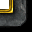Team Griffonrawl Trains Muay Thai and MMA fighters in Ohio.
Apex Steel Pipe - Buys and sells Steel Pipe.